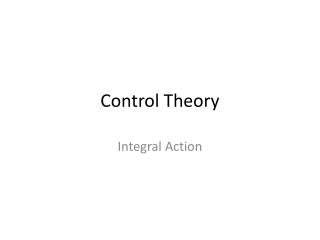DownloadDownload PresentationControl Theory

# Control Theory

Télécharger la présentation## Control Theory

- - - - - - - - - - - - - - - - - - - - - - - - - - - E N D - - - - - - - - - - - - - - - - - - - - - - - - - - -
##### Presentation Transcript

1. ControlTheory Integral Action

2. The formulas

3. Introduction

4. Steady state error of the servoproblemto a step of size 1(r0=z0=0)? • 0 • 2Kc • + • None of the above [Default] [MC Any] [MC All]

5. Integral Action Whenan integrator is present in the loop: steady state error = 0? Idea! We addanintegral in the control action. The PI controllernow looks like (in the time domain): TI is the ‘reset time’: it’s a measure for the time over which this integral is ‘smeared out’. A smaller reset time gives a stronger influence over a shorter period of time. (So notice: to put the I action off, the reset time should be infinity!)

6. The TF of the PI controller • Has a zero in zero and no poles • Has a zero in zero and one pole • Has a pole in zero and no zeros • Has a pole in zero and one zero [Default] [MC Any] [MC All]

7. Integral Action The PI controllernow looks like (in the Laplace domain):

8. First example

9. Example 1: The TF of the closed loop • Is of first order • Is of second order and onepole lies in 0 • Is of second order and contains a zero in 0 • Is of second order and contains a zero • Is of third order [Default] [MC Any] [MC All]

10. First example Calculate the steady state error.

11. Secondexample TakeKc=1 GROUP TASK: Canyou make the polescriticallydamped?

12. Example 1: The TF of the closed loop • No • The poles are alwayscriticallydamped • Yes, forTI = 0,75 • Yes, forTI = 7,5 • Yes, forTI = 10 [Default] [MC Any] [MC All]

13. Will I have overshoot in the step response forthatchoice of TI? • Yes • No [Default] [MC Any] [MC All]

14. Second example (Kc= 1,TI = 7.5)

15. Secondexample (Kc= 1)